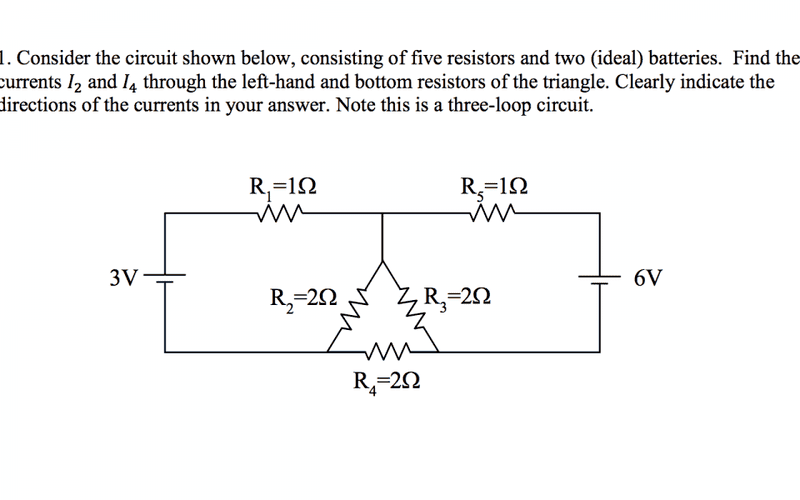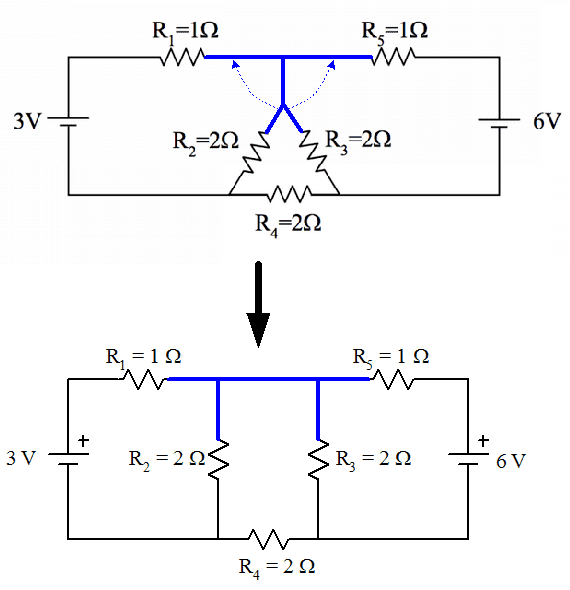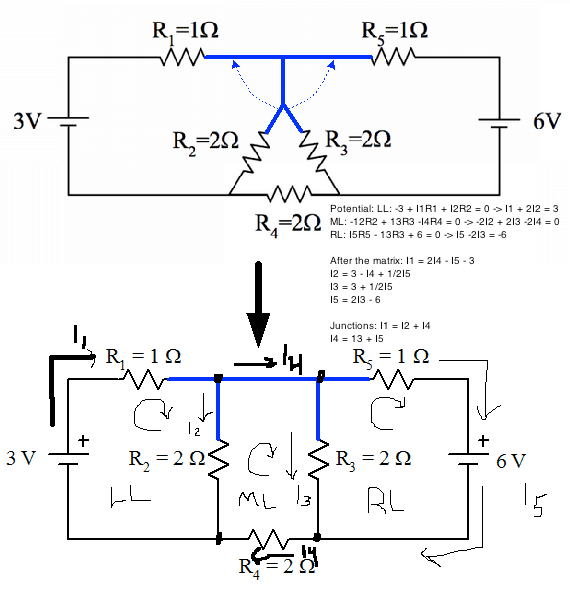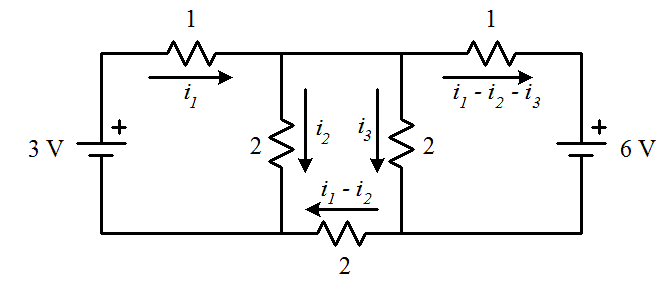# 3 loop circuit, 5 resistors, 2 batteries

## Homework Statement

Full problem statement and diagram is attached.

## Homework Equations

Kirchhoff's rules, ohm's law

## The Attempt at a Solution

So I presume that what I'll be looking at here is the use of Kirchhoff's Rules and Ohm's Law. I do not know how to approach this as I have never come across a problem involving a triangular loop. Are the resistors in series in this loop? They must be, but what does that give me? Can I collapse all three of them into one resistor? Would that benefit me? Also, I've split I1 at the top of the middle wire, giving me I3 going down and splitting up again around the triangle, giving me I5 going down through R2, and a new current I4 going through R3, as well as I2 going through R5 and continuing throughout the right loop. Is this correct?

These are unfinished obviously as I'm unsure of how to proceed, but I have: LeftLoop: -I1R1 - I3R3 + 3V = 0
MiddleLoop: I5R2 - I4R3 ... ? = 0
RightLoop: I4R3 - I2R5 - 6V = 0

I've chosen all loops to be clockwise. Please get back to me, appreciate ya

#### Attachments

•Physics.png
36 KB · Views: 2,280

I might be missing something, but there does not seem to be any way to replace the loop by a single resistor.

You will have to solve using variables - if you use current variables, you'll have only three unknowns (How? Think KJL).

If you use potential variables, you'll have two unknowns, one potential, for say, the top of the triangular loop, and one for either side, and at the same time assign a zero potential at the third end of the triangular loop.

Hope this helps.

•JesseJC
I might be missing something, but there does not seem to be any way to replace the loop by a single resistor.

You will have to solve using variables - if you use current variables, you'll have only three unknowns (How? Think KJL).

If you use potential variables, you'll have two unknowns, one potential, for say, the top of the triangular loop, and one for either side, and at the same time assign a zero potential at the third end of the triangular loop.

Hope this helps.
What do you mean by KJL? So I need to use more than just Kirchhoff's Rules and Ohm's law to deal with the triangular loop?

Junction Law - and yes.

Junction Law - and yes.
What is going on at R4?

SammyS
Staff Emeritus
Homework Helper
Gold Member
What do you mean by KJL? So I need to use more than just Kirchhoff's Rules and Ohm's law to deal with the triangular loop?
Kirchoff's Rules along with Ohm's Law is really all you need.

(Posting the figure in the thread.)gneill
Mentor
Don't let the triangular shape fool you. How components are arranged on the page is more art than substance. What matters is the topology of the circuit: what is connected to what.

Note that resistors R1, R2, R3, and R5 all share a node. It doesn't matter if a wire forks or slants or bends, as long as there's a contiguous conducting path that is wire it is all the same node. So if you want you can "straighten out" that triangular arrangement so long as the component connections are all made to the same nodes. In the figure below the wiring shown in blue comprises a single node. You're free to move connections to that node to any place on the node (you can "slide" connections along the wire path).•JesseJC
Don't let the triangular shape fool you. How components are arranged on the page is more art than substance. What matters is the topology of the circuit: what is connected to what.

Note that resistors R1, R2, R3, and R5 all share a node. It doesn't matter if a wire forks or slants or bends, as long as there's a contiguous conducting path that is wire it is all the same node. So if you want you can "straighten out" that triangular arrangement so long as the component connections are all made to the same nodes. In the figure below the wiring shown in blue comprises a single node. You're free to move connections to that node to any place on the node (you can "slide" connections along the wire path).
View attachment 97046
I've put all of the currents with their coefficients into a matrix and I've solved them, ending up with I2 = 1/2(2I5 + 3) and I4 = -(I5 +3)/2, which are the same. I just need to find one current and I'm golden, am I too dense? Where can I now apply Ohm's law?

Last edited:
gneill
Mentor
I've put all of the currents with their coefficients into a matrix and I've solved them, ending up with I2 = 1/2(2I5 + 3) and I4 = -(I5 +3)/2, which are the same. I just need to find one current and I'm golden, am I too dense? Where can I now apply Ohm's law?
We'll need to see your work in more detail to verify what you've found. If you can, take one of the circuit diagrams and draw in the currents that you're referencing; The directions that you assume for currents determines the signs of terms in the equations you form. Then write out the equations that you've obtained from the circuit.

Don't let the triangular shape fool you. How components are arranged on the page is more art than substance.
Does this make a difference? Won't we be using the same equations?

gneill
Mentor
Does this make a difference? Won't we be using the same equations?
Yes, but unfamiliar component layouts have a tendency to cause doubt and confusion about how to write the equations until students get a handle on "topology versus geography". This comes with practice. Realizing that the layout of components on a page does not affect circuit behavior is an important idea to internalize. Knowing that you can move component connections on a node can be useful in circuit simplifications, too, when it comes to recognizing parallel components and so forth.

•Qwertywerty
We'll need to see your work in more detail to verify what you've found. If you can, take one of the circuit diagrams and draw in the currents that you're referencing; The directions that you assume for currents determines the signs of terms in the equations you form. Then write out the equations that you've obtained from the circuit.Hopefully this illustrates whatever issue I'm having a bit better. I feel like I'm missing something very obvious.

gneill
Mentor
Can you write out your equations please? Their resolution on the image is not great. I think I can spot several issues there, but it's not easy to quote lines in an image.

epenguin
Homework Helper
Gold Member
It seems to me you get if not a slight simplification at least one thing less to think about by replacing the two batteries of 3 and 6V by just one of 3V on the right.
This would be okay in a simple circuit e.g. a single cycle of batteries and resistors in series. I couldn't immediately convince myself this would hold for a circuit with all these internal connections like this one. But I think it's OK. However complex the circuit, any path you trace between one battery and the other is going to have the same current and the same potential drops if at its ends there is 3V and 0V as when these ends are 6V and 3V. But maybe somebody else could make this clearer.

If you do it with one battery it is a bit easier to see. In fact you can do it with just the elementary formulae for series and parallel resistors. Though no doubt you should master the circuit techniques being talked about so far. I am getting I2 = 4/5 A, I4 = 3/5 A - subject to confirmation.

•JesseJC
SammyS
Staff Emeritus
Homework Helper
Gold Member
It seems to me you get if not a slight simplification at least one thing less to think about by replacing the two batteries of 3 and 6V by just one of 3V on the right.
This would be okay in a simple circuit e.g. a single cycle of batteries and resistors in series. I couldn't immediately convince myself this would hold for a circuit with all these internal connections like this one. But I think it's OK. However complex the circuit, any path you trace between one battery and the other is going to have the same current and the same potential drops if at its ends there is 3V and 0V as when these ends are 6V and 3V. But maybe somebody else could make this clearer.

If you do it with one battery it is a bit easier to see. In fact you can do it with just the elementary formulae for series and parallel resistors. Though no doubt you should master the circuit techniques being talked about so far. I am getting I2 = 4/5 A, I4 = 3/5 A - subject to confirmation.
Using one battery in this simple way is an over-simplification.

gneill
Mentor
Eliminating one of the sources and changing the other is not a valid approach. But you can suppress one at a time and use superposition. That is, find all the currents due to one battery alone, then find all the currents due to the other battery alone, then sum their contributions. That is a valid analysis approach.

SammyS
Staff Emeritus
Homework Helper
Gold Member
Eliminating one of the sources and changing the other is not a valid approach. But you can suppress one at a time and use superposition. That is, find all the currents due to one battery alone, then find all the currents due to the other battery alone, then sum their contributions. That is a valid analysis approach.
Superposition does explain how epenguin could get I4 correct but not I2 .

An alternate way to use superposition is to superimpose the results of the following two set-ups.
Use a single 3 V source at the position of the 6 V source.
Use two 3 V sources giving a symmetric circuit. With this symmetric circuit, I4 is zero.​

Can you write out your equations please? Their resolution on the image is not great. I think I can spot several issues there, but it's not easy to quote lines in an image.

Potential: LL: -3 + I1R1 + I2R2 = 0 -> I1 + 2I2 = 3

ML: -12R2 + 13R3 -I4R4 = 0 -> -2I2 + 2I3 -2I4 = 0

RL: I5R5 - 13R3 + 6 = 0 -> I5 -2I3 = -6

After the matrix: I1 = 2I4 - I5 - 3

I2 = 3 - I4 + 1/2I5

I3 = 3 + 1/2I5

I5 = 2I3 - 6

Junctions: I1 = I2 + I4

I4 = 13 + I5

epenguin
Homework Helper
Gold Member
Eliminating one of the sources and changing the other is not a valid approach. But you can suppress one at a time and use superposition. That is, find all the currents due to one battery alone, then find all the currents due to the other battery alone, then sum their contributions. That is a valid analysis approach.

Ah, that was an over-extrapolation of the simplest cases - I knew I should have analysed it mathematically that was not feeling up to it at this time. But I did feel uneasy.

It seems quite a good thing to do for this particular case - because of its symmetry - when you have done it for one battery, you have almost immediately the currents for the other battery!

gneill
Mentor
Potential: LL: -3 + I1R1 + I2R2 = 0 -> I1 + 2I2 = 3

ML: -12R2 + 13R3 -I4R4 = 0 -> -2I2 + 2I3 -2I4 = 0
From your diagram the current through R4 is going from right to left. Since your "KVL walk" is clockwise around the loop, the walk direction is the same as the current direction. So you should see a potential drop through R4. You have the R4 term as a negative, corresponding to a potential rise if you're summing drops (which, judging by the other terms is the case). So fix the sign of the ##I4 R4## term.
RL: I5R5 - 13R3 + 6 = 0 -> I5 -2I3 = -6
The other two KVL loop equations look okay (but watch for using digit "1" instead of capital "I" for currents! That 12⋅R2 and 13⋅R3 had me confused for a minute).
After the matrix: I1 = 2I4 - I5 - 3

I2 = 3 - I4 + 1/2I5

I3 = 3 + 1/2I5

I5 = 2I3 - 6
I'm not sure what this "matrix" step is, but you seem to have ended up with four equations in five unknowns, up from the three equations from KVL.
Junctions: I1 = I2 + I4

I4 = 13 + I5

Okay, not bad. You may want to create another node equation that recognizes the fact that your two "junctions" at the center top of the circuit actually comprise one node: the two junctions are connected by wire, so they are the same node. That equation would be:

I1 - I2 - I3 - I5 = 0.

That is, I1 flows into the node while I2, I3, and I5 flow out of the node. Since your I4 between the two junctions is actually a current internal to the node and never leaves the node, you can ignore it when writing KCL for the node.

If I may make a suggestion that could save you some effort, there is a way to label currents on your circuit that will reduce the number of unknowns that you have to deal with. The method involves only adding new currents (variables) when necessary as you proceed to label currents on the diagram.

So for example if you start by inserting I1 as you have it, then proceed to the top central node you'll see that there are three "outlets" there where currents fed by I1 can leave the node. In your diagram you have them as I2, I3 , and I5. The I4 current that you've put there is actually internal to the node. So rather than introducing four new currents, you can get away with adding two and making the last one a combination of the others. So leave I2 and I3 as you have them but replace I5 with I1 - I2 - I3, since your original I1 flows into the node and I2 and I3 are "tapped off" before reaching the last exit through resistor R5.

When you get to the bottom of the circuit, what you've labeled as I4 flowing through R4 can be made up from the existing currents flowing into the node, namely I3 and I1 - I2 - I3 (formerly I5). That makes that current total I1 - I2. What you get in the end is three currents rather than five to deal with:So when you write your loop equations you'll end up with exactly three equations in three unknowns, and you won't need to write separate junction equations (you've effectively incorporated them into the drawing's current labels).

•JesseJC
epenguin
Homework Helper
Gold Member
Did you get an answer for this?

Is I2 = 1.2 A (downwards), I4 = 0.6 A (L → R) ?

Last edited: